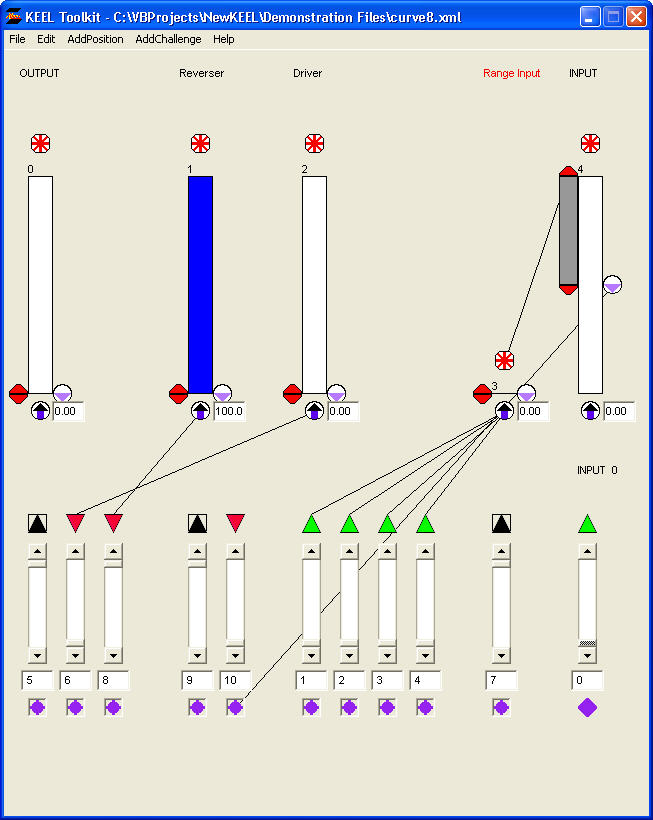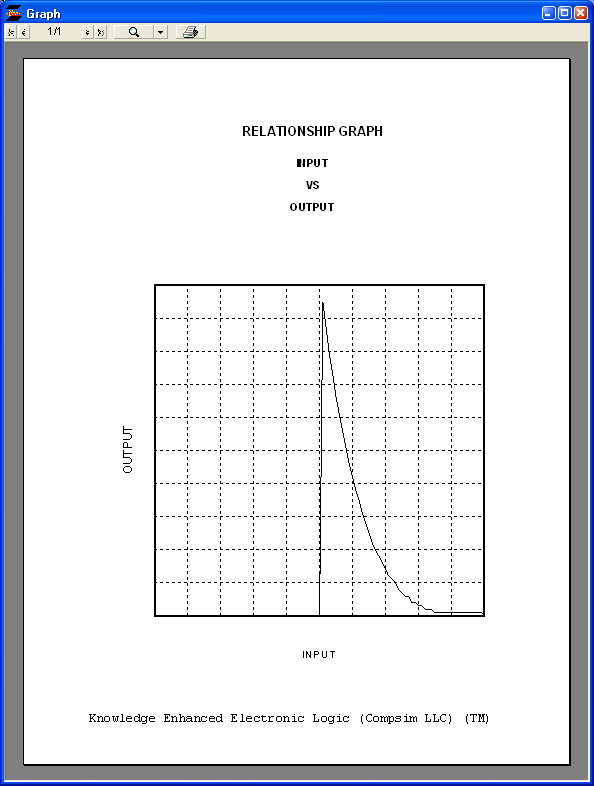Curve 8

curve8.xml

Concept

This is a delayed version of Curve 4 with an additional blocker that blocks the output until the point in time where the curve starts.

This is accomplsihed by using the clipper of the "INPUT" to define the range across where the curve is executed. In this case, the lower clipper is set at 50% and the upper clipper is set at 100%. The clipper is used to drive the importance of the "Range Input". The "Range Input" is then used to drive the "Driver" by multiplying its impact 4 times. Refer to the Curve 4 discussion.

The second part of this design is the use of the "INPUT" threshold to block the "OUTPUT" before the bottom of the "INPUT" clipper. To accomplish this, the "INPUT" threshold is set to the same value as the lower clipper of the "INPUT". The threshold is then run through a "Reverser" to invert its impact. Thus, when the modified value of the "INPUT" is lower than the threshold, the output of the "Reverser" is 100%, thus blocking the "OUTPUT". When the modified value of the "INPUT" is above the threshold, the output of the "Reverser" is 0%, thus having no impact on the "OUTPUT".

DesignOutput